# Intuitive Z-plane: Part 5 – Conclusion

If you’ve read through the first four parts of this series, then you’re already at a point where you can intuitively understand what’s going on. We just have a couple of details to take care of before finishing off.

Firstly, the plots showing the zeros and poles in the figures you’ve been looking at plots of the “Z-plane” or “Complex-plane“. As I said at the start, we’re only trying to get to an intuitive understanding of these plots – so I’m not going to get into complex numbers, or even much math (apart from what you’ll see below… which isn’t very complicated, and avoids complex numbers).

When I’m developing a new DSP algorithm, I use an application called Max from cycling74.com. Figure 1 shows a screenshot from Max, where I’m using an object to calculate the biquad coefficients to make a low pass filter, as you can see. I’ve then connected the output of that object (it looks like a magnitude response) to a Z-plan representation that shows me the same thing in a different way.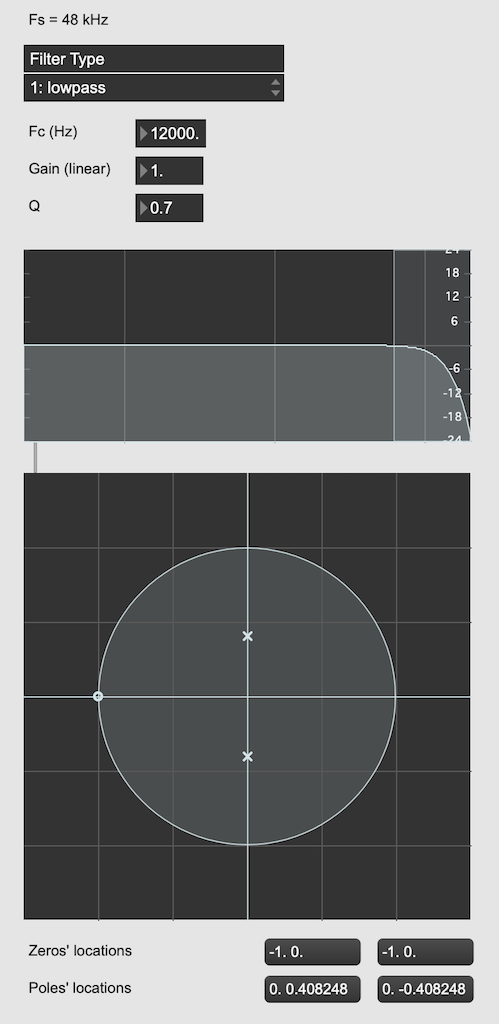Figure 1: The top plot shows the magnitude response of the filter. The bottom plot shows the Z-plane representation of the same filter.

You may notice that this plot has two poles, one at (0, 0.408) and the other at (0, -0.408). In fact there are two zeros there as well, but they’re situated in the same place, on “on top” of the other, at (-1, 0). This is always true for a biquad – there are always two zeros and two poles. Sometimes, they’re located in the same place, sometimes not, sometimes they’re placed symmetrically, sometimes not, depending on the filter, as we’ll see below.

Let’s look at that Z-plane representation in 3-dimensions: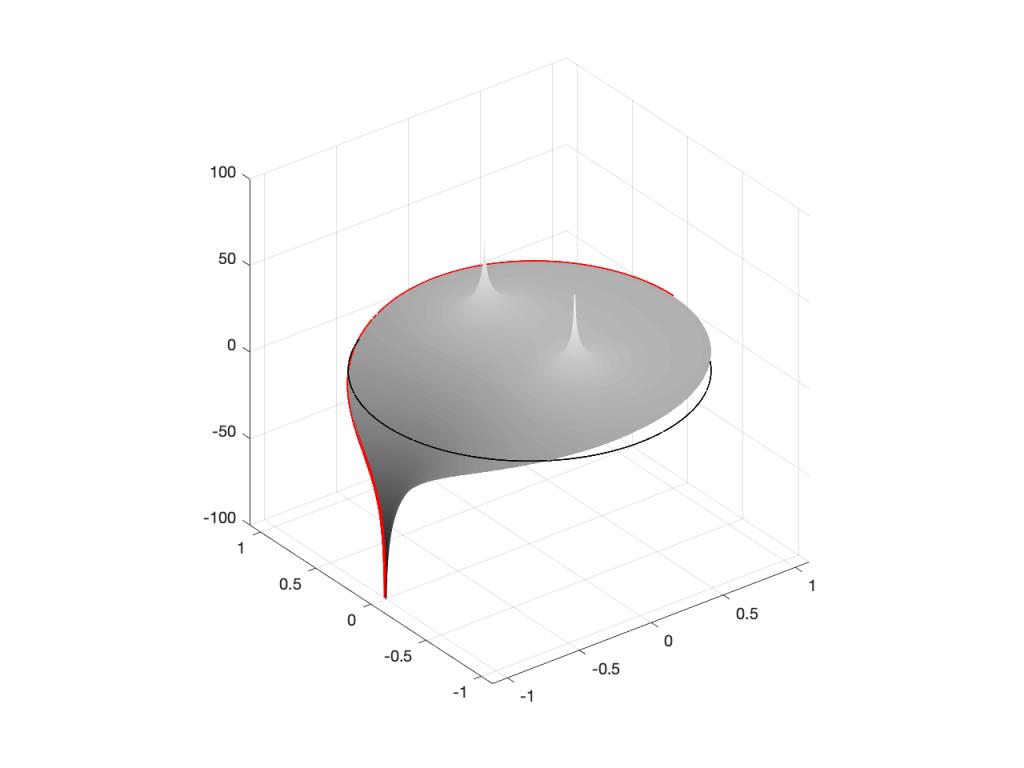Figure 2: A 3D view of the Z-plane representation of the filter shown in Figure 1.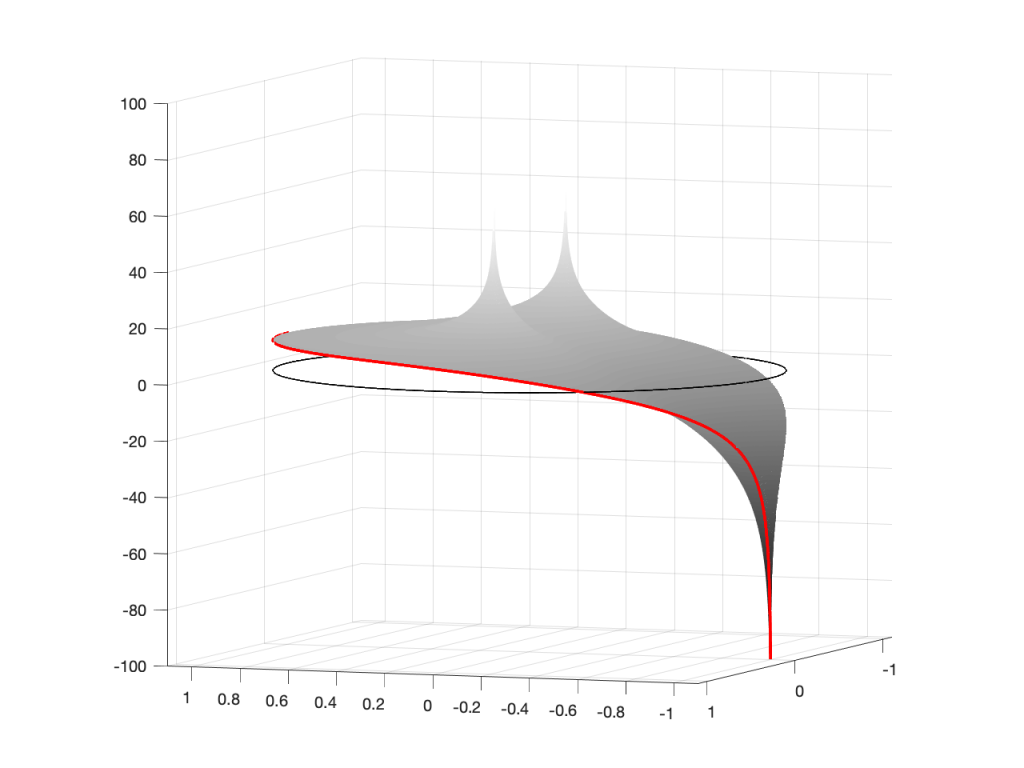Figure 3: The same plot as shown in Figure 2, rotated to show the back of the plot.

So, as you would now expect, the poles pull up the edge of the circle, and the zeros (both in the same place) pull down, giving the red line the height that it has.

Now, think back to this Figure from earlier in the series:

If you therefore look at Figure 3, which is like looking at Figure 4 from the top, you’ll notice that the height of the red line (the edge of the circle is high on the left (in the low frequencies) and drops as you go to the right (the high frequencies). This is the magnitude response that’s shown on the top of Figure 1. The only difference is that it’s on a linear scale instead of a logarithmic scale, so the shape looks a little weird.

Let’s do another one: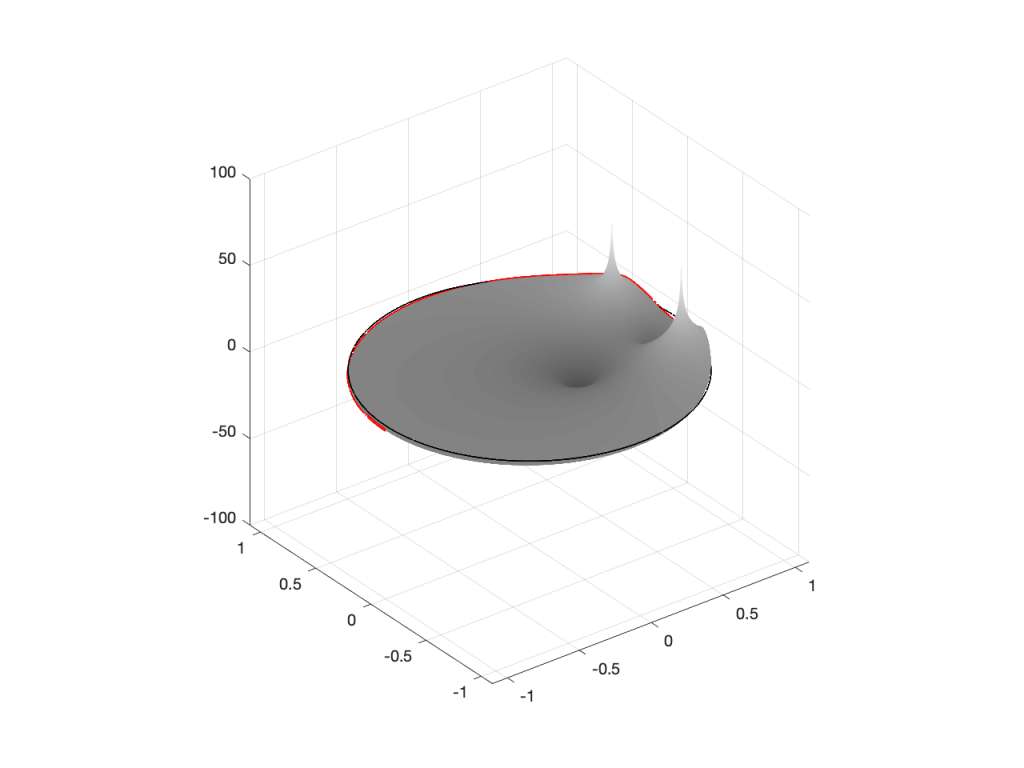Figure 6: A 3D view of the Z-plane representation of the filter shown in Figure 5.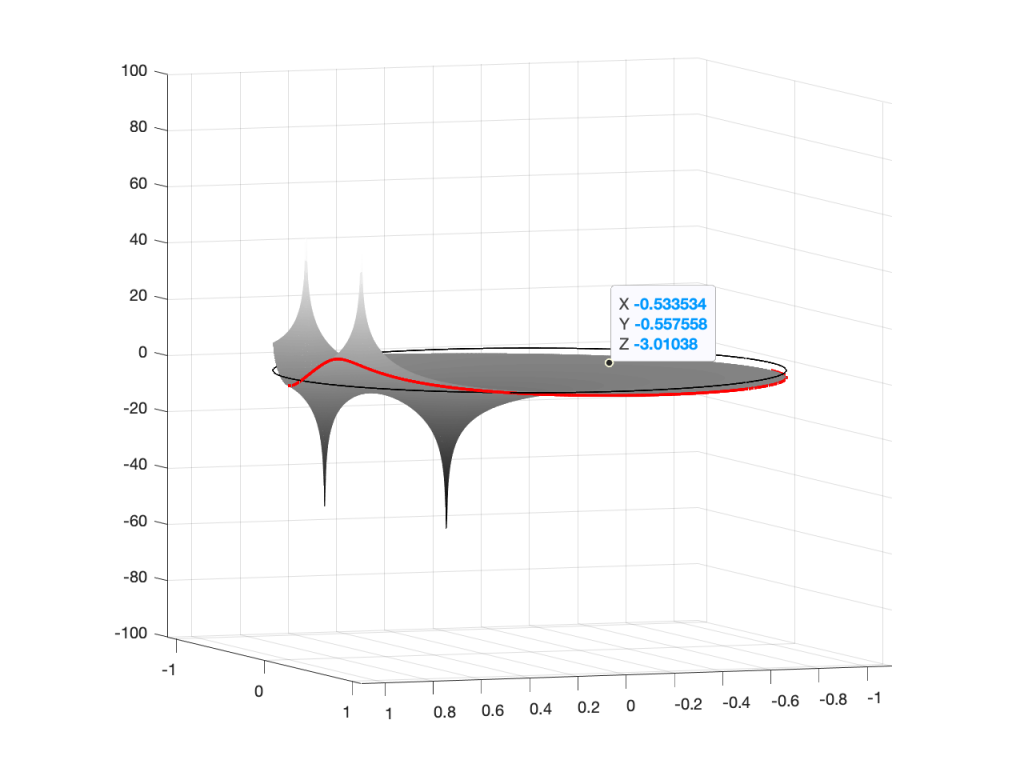Figure 7: The same plot as shown in Figure 6, rotated to show the back of the plot.

Hopefully, now you are able to look at a Z-plane representation of a filter and think about the effect of the poles and zeros on the edge of the circle, and therefore get a rough idea of the magnitude response of the filter…

If not, I apologize for wasting your time. On the other hand, if you’re in a life-threatening situation, this knowledge probably wouldn’t help you anyway… Very few people have gotten a critical injury in a biquad accident.

## How I did it

If you want to make these plots for yourself, the math is pretty simple.